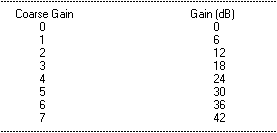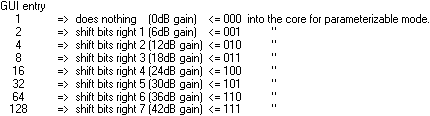# AR# 16420

|

## 描述

Several errors exist in the Digital Down Converter (DDC) data sheet:

The errors and their corrections are explained below:

## 解决方案

1. The section on DDS Tuning (pg 6) presents an example illustrating the calculation of the DDS output frequency. Equation 3 shows the calculation for "fout", and the result is shown as "27.939677 MHz".

Correction: The answer should be given in Hz, not MHz. The 1000 in this equation is the phase increment.

(120 MHz x 1000) divided by (2 raised to 32) = 27.939 Hz (not 27.939 MHz)

2. The last paragraph in the DDS Tuning section (pg 6) states that the "phase increment value can be computed using this information together with the core clock frequency and EQ.(4)".

Correction: The core sample rate should be used, not the core clock frequency. The "fs" in EQ.(4) represents the sample rate.

3. Under the Decimating Half-Band Filter title on page 25, a message reports: "Error! Reference source not found". The same message appears under Figure 47.

Correction: This paragraph and Figure 47 are describing the coefficient file that corresponds to the graph in Figure 49.

4. Figure 54 shows a coefficient file (.coe) for the PFIR H(z) filter. This coefficient file is not symmetrical. Entering this .coe file into the DDC GUI will cause an error.

Correction: The coefficients must be updated so that they are symmetrical before the GUI will accept them.

5. Table 4 explains how to set the CIC Gain. This table does not match the settings that appear on the DDC GUI screen when the core is built.

The table's "coarse gain" and "gain" columns contain the values shown below:However, the GUI screen selections are [1, 2, 4, 8, 16, 32, 64, 128 ]. These values do not match either column.

Correction: The GUI selections will produce the same resulting gain, but the data sheet does not explain how the numbers are related. The gain is implemented by right-shifting the bits as follows:AR# 16420

Type 综合文章
People Also Viewed